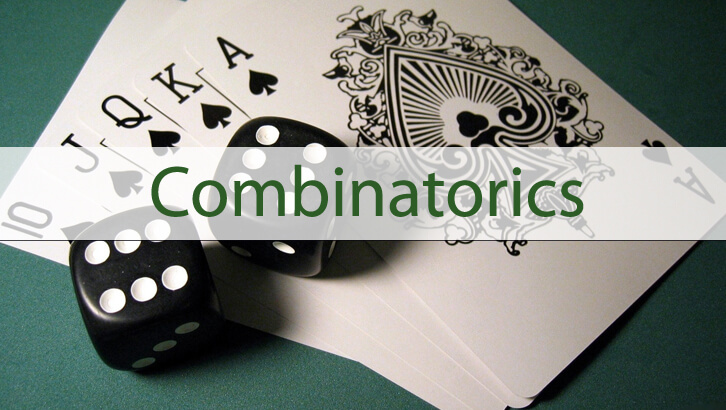# Permutation and Combination (with a touch of Number Theory)

Have given below a few questions on Counting that also include a bit of Number Theory. These types of questions are commonly tested.

1. a, b, c are three distinct integers from 2 to 10 (both inclusive). Exactly one of ab, bc and ca is odd. abc is a multiple of 4. The arithmetic mean of a and b is an integer and so is the arithmetic mean of a, b and c. How many such triplets are possible (unordered triplets)

2. A seven-digit number comprises of only 2’s and 3’s. How many of these are multiples of 12?

3. How many factors of 2^5 * 3^6 * 5^2 are perfect squares?

4. How many factors of 2^4 * 5^3 * 7^4 are odd numbers?

5. A number when divided by 18 leaves a remainder 7. The same number when divided by 12 leaves a remainder n. How many values can n take?

## One comment

1.tulsi.. (cake-walker..) !! says:

Is the answer for the 4th Question, 20 Factors?